# Absolute Value Equations and Inequalities

#### Whats is Absolute value?

Absolute value (or Modulus) represented by |x| of a real number x is the non-negative value of x without regard to its sign.

For example:

Absolute value of -3 => |-3| = 3
Absolute value of 7 => |7| = 7

#### Absolute value as distance?

We can also think of absolute value of a number as its distance from zero. For example, consider this number line,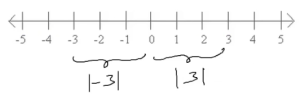Here, the integer 3 is at a distance of 3 units from number 0. Similarly number -3 is also at distance of 3 units from 0. Since distance is always positive, we can say that,

The Distance of 3 from 0 => |3| = 3 & The Distance of -3 from 0 => |-3| = 3

Now, if |x| = 3, then x is at a distance of 3 units from 0. From the number line, we get that the absolute of x = 3 or x = -3

In general, if |x| = a => x = a or x = -a. (Since x is at a distance of ‘a’ units from 0).

#### If |x| = a is the distance of x from 0, then what is |x-a|?

Distance of x from a number ‘a’ on the number line can be represented by |x-a|.

1. Solve the equation |x-2| = 3.
|x-2| = 3 implies x is at a distance of 3 units from 2. Representing this on the number line,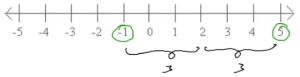The numbers on the number line which are at a distance of 3 units from 2 are 5 and -1. Therefore, x = 5 or x = -1 are the values of the equation |x-2| = 3.

2. Solve the inequality |x-2| < 3
|x-2| = 3 implies x is at a distance less than 3 units from 2. Representing this on the number line,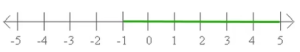From the number line, we see that all the points between -1 and 5 are at a distance less than 3 units from 2. Hence, the values taken by x is given by -1 < x < 5.

#### If you know what is |x| = a, can you explain what is meant by |x-a| + |x-b| ?

From our understanding of absolute value so far, we know that
|x – a| represents the distance of x from a. Similarly |x – b| represents the distance of x from b. Implying that |x-a| + |x-b| is the sum of the distances of x from both a and b! :). This is evident from the number line,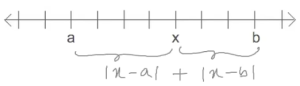Solve for x, |x+2| + |x-3| = 7
=> |x-(-2)| + |x-3| = 7 i.e we have to calculate the sum of the distances of x from -2 and 3.

Representing this on the number line,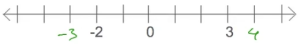From the image, we see that 4 is at distance of 1 unit from 3 and 6 units from -2. Hence, the sum of the distances of 4 from 3 and -2 is 7.
Similarly the sum of the distances of -3 from 4 and 3 is 7.

Therefore, x = 4 or x = -3 are the values of the equation |x+2| + |x-3| = 7.

#### When is the distance of x from ‘a’ and ‘b’ minimum?

We know that the sum of the distances of x from a and b is represented in terms of absolute value by |x – a| + |x – b|.

Now, |x – a| + |x – b| is minimum when x lies between a and b. The minimum value is given by |b-a|.

What is the minimum value of |x+2| + |x-3|?
=> Minimum value = |3-(-2)| = 5, which occurs for -2 ≤ x ≤ 3

## Solving Absolute Value Equations

### Math Tricks Workout

Please do try our android app – Math Tricks Workout. The app is developed to improve mental arithmetic using a series of left to right fast math workouts.

Scan the QR code below or click on it for more details.